PDE Rendering VI

LabVIEW 2018 Help

Edition Date: March 2018
Part Number: 371361R-01
View Product InfoLabVIEW 2016 HelpLabVIEW 2017 HelpLabVIEW 2018 HelpLabVIEW 2019 HelpLabVIEW 2020 Help

Owning Palette: Partial Differential Equations VIs

Requires: Full Development System

Renders the solution of the partial differential equation. You must manually select the polymorphic instance to use.

Use the pull-down menu to select an instance of this VI.

 Select an instance 2D Helmholtz (Rectangular domain)2D Helmholtz (Polygonal domain)1D Heat2D Heat (Rectangular domain)1D Wave2D Wave (Rectangular domain)

2D Helmholtz (Rectangular domain)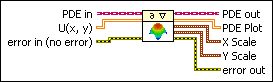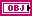PDE in is the class that stores the data of the equation.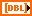U(x, y) specifies the solution of the equation.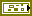error in describes error conditions that occur before this node runs. This input provides standard error in functionality.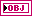PDE out returns PDE in unchanged.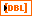PDE Plot returns an intensity graph that renders U(x, y).X Scale returns the offset and multiplier of the x-scale. X Scale returns the offset and multiplier of the PDE Plot x-scale.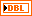Offset returns the offset of the x-scale.Multiplier returns the multiplier of the x-scale.Y Scale returns the offset and multiplier of y-scale. Y Scalereturns the offset and multiplier of the PDE Plot y-scale.Offset returns the offset of the y-scale.Multiplier returns the multiplier of the y-scale.error out contains error information. This output provides standard error out functionality.

2D Helmholtz (Polygonal domain)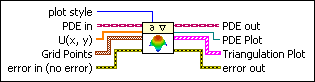plot style specifies how LabVIEW draws the solution for PDE Plot.

 0 Wireframe—Draws the solution with line segments that connect at Grid Points. 1 Polygons (default)—Draws the solution with filled geometry.PDE in is the class that stores the data of the equation.U(x, y) specifies the solution of the equation in Grid Points from the PDE Solver VI.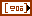Grid Points specifies the grid points for the equation.X specifies the x-coordinate of Grid Points.Y specifies the y-coordinate of Grid Points.error in describes error conditions that occur before this node runs. This input provides standard error in functionality.PDE out returns PDE in unchanged.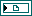PDE Plot returns a reference to a 3D scene object. Wire this output to the 3D picture control to view the solution.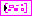Triangulation Plot returns an XY graph that illustrates triangulation.error out contains error information. This output provides standard error out functionality.

1D Heat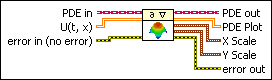PDE in is the class that stores the data of the equation.U(t, x) specifies the solution of the equation.error in describes error conditions that occur before this node runs. This input provides standard error in functionality.PDE out returns PDE in unchanged.PDE Plot returns an intensity graph that renders U(t, x).X Scale returns the offset and multiplier of the x-scale. X Scale returns the offset and multiplier of the PDE Plot x-scale.Offset returns the offset of the x-scale.Multiplier returns the multiplier of the x-scale.Y Scale returns the offset and multiplier of y-scale. Y Scalereturns the offset and multiplier of the PDE Plot y-scale.Offset returns the offset of the y-scale.Multiplier returns the multiplier of the y-scale.error out contains error information. This output provides standard error out functionality.

2D Heat (Rectangular domain)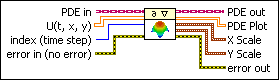PDE in is the class that stores the data of the equation.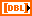U(t, x, y) specifies the solution of the equation.index (time step) specifies the index of the time step.

If index (time step) is negative or greater than # of t-points from the Define PDE Domain VI, LabVIEW renders the solution at final t. Otherwise, LabVIEW renders the solution at index (time step). The default is –1.error in describes error conditions that occur before this node runs. This input provides standard error in functionality.PDE out returns PDE in unchanged.PDE Plot returns an intensity graph that renders U(t, x, y) at index (time step).X Scale returns the offset and multiplier of the x-scale. X Scale returns the offset and multiplier of the PDE Plot x-scale.Offset returns the offset of the x-scale.Multiplier returns the multiplier of the x-scale.Y Scale returns the offset and multiplier of y-scale. Y Scalereturns the offset and multiplier of the PDE Plot y-scale.Offset returns the offset of the y-scale.Multiplier returns the multiplier of the y-scale.error out contains error information. This output provides standard error out functionality.

1D WavePDE in is the class that stores the data of the equation.U(t, x) specifies the solution of the equation.error in describes error conditions that occur before this node runs. This input provides standard error in functionality.PDE out returns PDE in unchanged.PDE Plot returns an intensity graph that renders U(t, x).X Scale returns the offset and multiplier of the x-scale. X Scale returns the offset and multiplier of the PDE Plot x-scale.Offset returns the offset of the x-scale.Multiplier returns the multiplier of the x-scale.Y Scale returns the offset and multiplier of y-scale. Y Scalereturns the offset and multiplier of the PDE Plot y-scale.Offset returns the offset of the y-scale.Multiplier returns the multiplier of the y-scale.error out contains error information. This output provides standard error out functionality.

2D Wave (Rectangular domain)PDE in is the class that stores the data of the equation.U(t, x, y) specifies the solution of the equation.index (time step) specifies the index of the time step.

If index (time step) is negative or greater than # of t-points from the Define PDE Domain VI, LabVIEW renders the solution at final t. Otherwise, LabVIEW renders the solution at index (time step). The default is –1.error in describes error conditions that occur before this node runs. This input provides standard error in functionality.PDE out returns PDE in unchanged.PDE Plot returns an intensity graph that renders U(t, x, y) at index (time step).X Scale returns the offset and multiplier of the x-scale. X Scale returns the offset and multiplier of the PDE Plot x-scale.Offset returns the offset of the x-scale.Multiplier returns the multiplier of the x-scale.Y Scale returns the offset and multiplier of y-scale. Y Scalereturns the offset and multiplier of the PDE Plot y-scale.Offset returns the offset of the y-scale.Multiplier returns the multiplier of the y-scale.error out contains error information. This output provides standard error out functionality.

PDE Rendering Details

Before you use the PDE Rendering VI, use the PDE Solver VI to solve the equation.

The following block diagram illustrates how to set the scale offset and multiplier of PDE Plot by X Scale and Y Scale.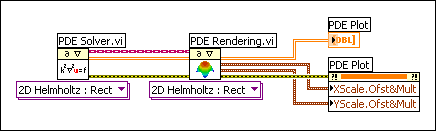If you do not set the scale offset and multiplier, LabVIEW uses the default settings of the intensity graph.

Examples

Refer to the following VIs for examples of using the PDE Rendering VI:

• PDE Flexible Element VI: labview\examples\Mathematics\Differential Equations - PDE
• PDE String Vibration VI: labview\examples\Mathematics\Differential Equations - PDE
• PDE Thermal Distribution VI: labview\examples\Mathematics\Differential Equations - PDE